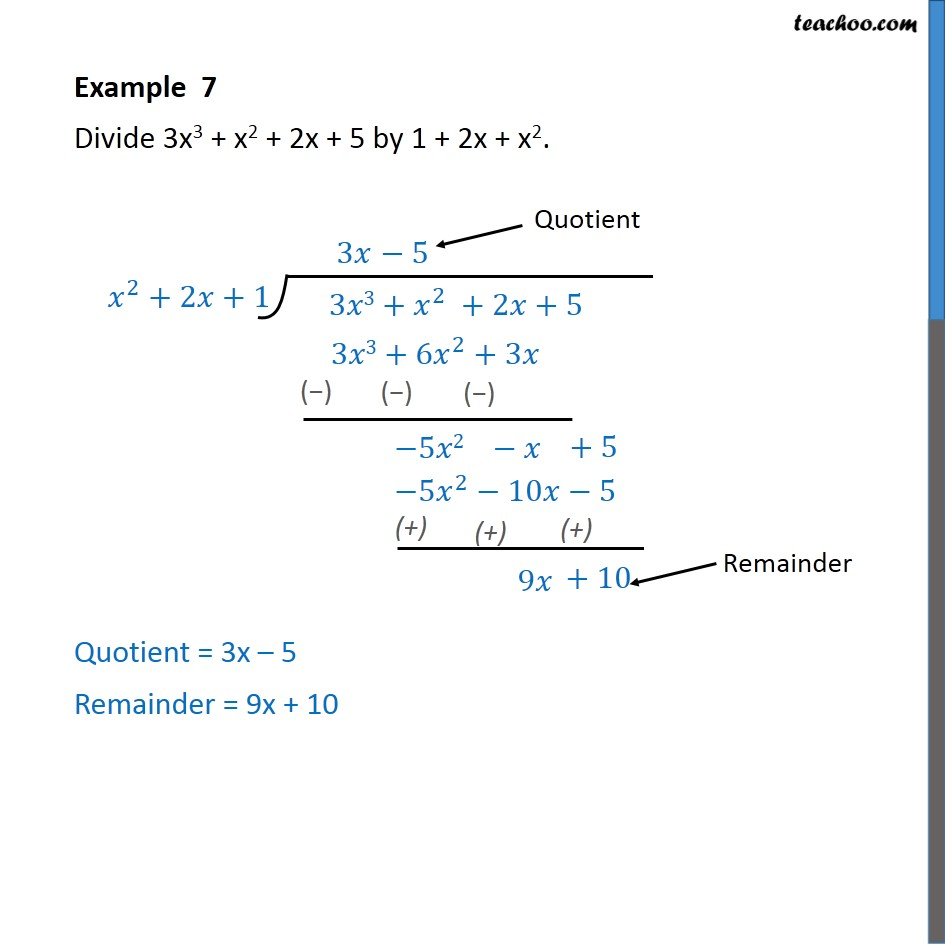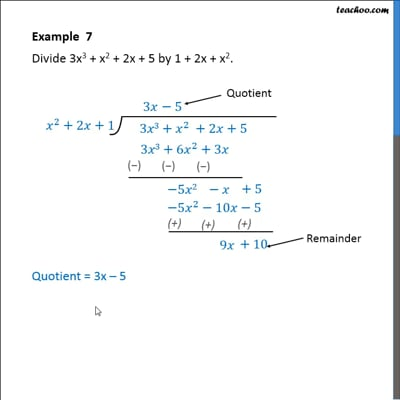Examples

Chapter 2 Class 10 Polynomials
Serial order wiseThis video is only available for Teachoo black users

Solve all your doubts with Teachoo Black (new monthly pack available now!)

### Transcript

Example 7 Divide 3x3 + x2 + 2x + 5 by 1 + 2x + x2. We follow these steps 1. Since we have 3x3, we multiply by 3x 2. Thus, the remainder will be difference of 3x3 + x2 + 2x and 3x3 + 6x2 + 3x 3. Which comes out to be -5x2 - x 4. Now, we have -5x2, so we multiply with -5 5. So, the remainder will be difference of -5x2 - x + 5 and -5x2 -10x - 5 6. Which comes out to be 9x + 10 Therefore, Quotient = 3x – 5 Remainder = 9x + 10